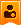##账号 自动登录 找回密码 密码 注册

# [fx-9860/9750] [fx-CG10/20/50] C.Basic cnCalc资讯站发表于 2020-5-15 08:27:40 | 显示全部楼层
 CalcLoverHK 发表于 2020-5-15 01:29 最近的话你可以帮我（我还要上网课到5/27），感谢！ 可以发表于 2020-5-15 13:03:54 | 显示全部楼层
 期待新版本早点更新解决。发表于 2020-5-20 15:21:10 | 显示全部楼层
 1.45 Build 19版现在发布了 修复了使用Break命令从Try〜TryEnd内部逃避循环后[AC] / AcBreak不起作用的错误。 修复了导致系统错误的错误，使其成为堆栈错误。 更改了适用于当前VRAM的ReadGraph / WriteGraph / DotGet / DotPut命令的规范。 增加了Screen命令的V选项，以将绘图目标VRAM更改为文本VRAM /图形VRAM的撤退区域。 （格式）Screen.V   设置为默认VRAM。 （格式）Screen.VT   将文本VRAM的撤退区域更改为图形。 （格式）Screen.VG   将图形VRAM的撤退区域更改为图形。发表于 2020-5-21 13:56:18 | 显示全部楼层
 diaowinner 发表于 2020-5-13 20:34 应该添加＃CBCPLX的 大神，请问我这个开三次根号报错误，该具体怎么操作解决啊？发表于 2020-5-22 15:38:15 | 显示全部楼层

## emm……

 ty2015ty 发表于 2020-5-21 13:56 大神，请问我这个开三次根号报错误，该具体怎么操作解决啊？ 可以发给我代码吗？在你的三次根号公式前放#CBCPLX发表于 2020-5-27 08:59:46 来自手机 | 显示全部楼层
 又来个个bug修复版 :) 2.45 beta build20 9860G系列     (build20)   2020.5.21 -添加法文说明书（没啥用）     (build19)   2020.5.20         -在System(-1)选项增加9750GIII         　0:fx-9860G(SH3)           1:fx-9860G Slim(SH3)           2:fx-9860GII(SH3)           3:fx-9860GII(SH4A)           4:Graph35+EII(SH4A)           5:fx-9860GIII(SH4A)           6:fx-9750GIII(SH4A)         -System()指令样本程序更新了. (System_sample文件夹) 1.45 beta build20 for CG10/20/50/Graph90+E. https://pm.matrix.jp...CG145beta20.zip     (build20)   2020.5.21         -添加GRAPH90+E版         (格式) System(-1)          回车为 CG10/20/50          返回10 : CG10          返回20 : CG20          返回50 : CG50          返回90 : Graph90+E         -也更新了System（）的演示程序 (System_sample 文件夹) (common update)     (build20)   2020.5.21         -修复跳过缓存在If~ElseIf中不能运行.         -新增新的System（）选项以用于获取版本         (格式) System(-22)发表于 2020-6-1 10:06:40 | 显示全部楼层
 diaowinner 发表于 2020-5-22 15:38 可以发给我代码吗？在你的三次根号公式前放#CBCPLX 'ProgramMode:RUN Deg Norm 1 "[email protected][email protected][email protected]__<[email protected][email protected]__#E691_b=_#E6A1_1_#[email protected]__<[email protected]__#E691_b=_#E6A1_2_#E6A2_.e_<=_>b//6_#E691_b=_#E6A1_3_#E6A2_"? If =2 Then "[email protected]_ (kN)"?N "[email protected]_ (m)"?d "_#[email protected]_ (kN//m_#E5C3_)"?g "[email protected]_ (kPa)"?F If F=0 Then Prog "003.fa" "_#[email protected]__#E6A2_=1._#E6A1__#E6A4__#E6A2_=2._#E6A1__#E6A5__#E6A2_=3"?yIf y=1 Then N/(F-gd)->b "b_>=_ (m)":bDisps "a (m)="?a "_#E650_ (_dms_)"?p "_#E641_ (_dms_)"?B (a+d/tan (B-p))/3.5->b"b_>=_ (m)":bDisps ElseIf y=2 Then Sqrt(4(N/(F-gd))/pi)->d "d_>=_ (m)":dDisps ElseIf y=3 Then "l (m)"?l N/(l(F-gd))->b "b_>=_ (m)":bDisps "a (m)="?a "_#E650_ (_dms_)"?p "_#E641_ (_dms_)"?B (a+d/tan (B-p))/2.5->b"b_>=_ (m)":bDisps IfEnd Else "_#[email protected]__#E6A2_=1._#E6A1__#E6A4__#E6A2_=2._#E6A1__#E6A5__#E6A2_=3"?yIf y=1 Then N/(F-gd)->b "b_>=_ (m)":bDisps "a (m)="?a "_#E650_ (_dms_)"?p "_#E641_ (_dms_)"?B (a+d/tan (B-p))/3.5->b"b_>=_ (m)":bDisps ElseIf y=2 Then Sqrt(4(N/(F-gd))/pi)->d "d_>=_ (m)":dDisps ElseIf y=3 Then "l (m)"?l N/(l(F-gd))->b "b_>=_ (m)":bDisps "a (m)="?a "_#E650_ (_dms_)"?p "_#E641_ (_dms_)"?B (a+d/tan (B-p))/2.5->b"b_>=_ (m)":bDisps IfEnd IfEnd ElseIf =1 Then "[email protected]_ (kN)"?N "[email protected]_ (m)"?d "z (m)"?z "_#[email protected]_ (kN//m_#E5C3_)"?g "_Theta_ (_dms_)"?Theta "[email protected][email protected]_ (kPa)"?L If L=0 Then Prog "105.Pcz" "[email protected][email protected]_ (kPa)"?F "[email protected]_ (m)"?d "[email protected]_ (kPa)"?C If C=0 Then Prog "103.Pc" "_#[email protected]__#E6A2_=1._#E6A1__#E6A4__#E6A2_=2._#E6A1__#E6A5__#E6A2_=3"?yIf y=1 Then (N-2z(F-L)tan Theta)/(F-L-gd+C)->b "b_>=_ (m)":bDisps ElseIf y=2 Then (4z(F-L)tan Theta+Sqrt((4z(F-L)tan Theta)^<2>-4(N-(F-L)(2ztan Theta)^<2>)((pi(gd-C)/4)-(F-L)))/(2(pi(gd-C)/4)-(F-L))->D (4z(F-L)tan Theta-Sqrt((4z(F-L)tan Theta)^<2>-4(N-(F-L)(2ztan Theta)^<2>)((pi(gd-C)/4)-(F-L)))/(2(pi(gd-C)/4)-(F-L))->U "D_>=_ (m)":DDisps "D'_>=_ (m)":UDisps ElseIf y=3 Then "l (m)"?l (N/l-(2z(F-L)tan Theta)(1+(2z/tan Theta)/l))/((F-L)(1+(2ztan Theta)/l)-gd+C)->b "b_>=_ (m)":bDisps IfEnd Else "_#[email protected]__#E6A2_=1._#E6A1__#E6A4__#E6A2_=2._#E6A1__#E6A5__#E6A2_=3"?yIf y=1 Then (N-2z(F-L)tan Theta)/(F-L-gd+C)->b "b_>=_ (m)":bDisps ElseIf y=2 Then (4z(F-L)tan Theta+Sqrt((4z(F-L)tan Theta)^<2>-4(N-(F-L)(2ztan Theta)^<2>)((pi(gd-C)/4)-(F-L)))/(2(pi(gd-C)/4)-(F-L))->D (4z(F-L)tan Theta-Sqrt((4z(F-L)tan Theta)^<2>-4(N-(F-L)(2ztan Theta)^<2>)((pi(gd-C)/4)-(F-L)))/(2(pi(gd-C)/4)-(F-L))->U "D_>=_ (m)":DDisps "D'_>=_ (m)":UDisps ElseIf y=3 Then "l (m)"?l (N/l-(2z(F-L)tan Theta)(1+(2z/tan Theta)/l))/((F-L)(1+(2ztan Theta)/l)-gd+C)->b "b_>=_ (m)":bDisps IfEnd IfEnd Else "[email protected][email protected]_ (kPa)"?F "[email protected]_ (m)"?d "[email protected]_ (kPa)"?C If C=0 Then Prog "103.Pc" "_#[email protected]__#E6A2_=1._#E6A1__#E6A4__#E6A2_=2._#E6A1__#E6A5__#E6A2_=3"?yIf y=1 Then (N-2z(F-L)tan Theta)/(F-L-gd+C)->b "b_>=_ (m)":bDisps ElseIf y=2 Then (4z(F-L)tan Theta+Sqrt((4z(F-L)tan Theta)^<2>-4(N-(F-L)(2ztan Theta)^<2>)((pi(gd-C)/4)-(F-L)))/(2(pi(gd-C)/4)-(F-L))->D (4z(F-L)tan Theta-Sqrt((4z(F-L)tan Theta)^<2>-4(N-(F-L)(2ztan Theta)^<2>)((pi(gd-C)/4)-(F-L)))/(2(pi(gd-C)/4)-(F-L))->U "D_>=_ (m)":DDisps "D'_>=_ (m)":UDisps ElseIf y=3 Then "l (m)"?l (N/l-(2z(F-L)tan Theta)(1+(2z/tan Theta)/l))/((F-L)(1+(2ztan Theta)/l)-gd+C)->b "b_>=_ (m)":bDisps IfEnd Else "_#[email protected]__#E6A2_=1._#E6A1__#E6A4__#E6A2_=2._#E6A1__#E6A5__#E6A2_=3"?yIf y=1 Then (N-2z(F-L)tan Theta)/(F-L-gd+C)->b "b_>=_ (m)":bDisps ElseIf y=2 Then (4z(F-L)tan Theta+Sqrt((4z(F-L)tan Theta)^<2>-4(N-(F-L)(2ztan Theta)^<2>)((pi(gd-C)/4)-(F-L)))/(2(pi(gd-C)/4)-(F-L))->D (4z(F-L)tan Theta-Sqrt((4z(F-L)tan Theta)^<2>-4(N-(F-L)(2ztan Theta)^<2>)((pi(gd-C)/4)-(F-L)))/(2(pi(gd-C)/4)-(F-L))->U "D_>=_ (m)":DDisps "D'_>=_ (m)":UDisps ElseIf y=3 Then "l (m)"?l (N/l-(2z(F-L)tan Theta)(1+(2z/tan Theta)/l))/((F-L)(1+(2ztan Theta)/l)-gd+C)->b "b_>=_ (m)":bDisps IfEnd IfEnd IfEnd ElseIf =3 Then "[email protected]_ (kN_#E6AA_m)"?M "[email protected]_ (kN)"?N "[email protected]_ (m)"?d "_#[email protected]_ (kN//m_#E5C3_)"?g "_#[email protected]__#E6A2_=1._#E6A1__#E6A4__#E6A2_=2._#E6A1__#E6A5__#E6A2_=3"?yIf y=1 Then "l (m)"?l (-N+Sqrt(N^<2>+24gdlM))/(2gdl)->b (-N-Sqrt(N^<2>+24gdlM))/(2gdl)->B "b_>=_ (m)":bDisps "b_>=_ (m)":BDisps Prog "003.fa" Prog "102.Pk" Prog "368Pkmax" ElseIf y=2 Then N/(pigd/4)->u -8M/(pigd/4)->v "u =":uDisps "v =":vDisps Cbrt(-v/2+Sqrt((v/2)^<2>+(u/2)^(3)))+Cbrt(-(v/2)-Sqrt((v/2)^<2>+(u/2)^(3)))->D Imaginary((-1+Sqrt3)/2)(Cbrt(-v/2+Sqrt((v/2)^<2>+(u/2)^(3))))+(Cbrt(-(v/2)-Sqrt((v/2)^<2>+(u/2)^(3))))Imaginary((-1-Sqrt3)/2)->I Imaginary((-1-Sqrt3)/2)(Cbrt(-v/2+Sqrt((v/2)^<2>+(u/2)^(3))))+(Cbrt(-(v/2)-Sqrt((v/2)^<2>+(u/2)^(3))))Imaginary((-1+Sqrt3)/2)->J "D_>=_ (m)":DDisps "D_>=_ (m)":IDisps "D_>=_ (m)":JDisps Prog "003.fa" Prog "102.Pk" Prog "368Pkmax" ElseIf y=3 Then N/(gd)->u -6M/(gd)->v "u =":uDisps "v =":vDisps (v/2)^<2>+(u/2)^(3)->q Cbrt(-v/2+Sqrt(q))+Cbrt(-(v/2)-Sqrt(q))->b Imaginary((-1+Sqrt3)/2)(Cbrt(-v/2+Sqrt(q)))+(Cbrt(-(v/2)-Sqrt(q)))Imaginary((-1-Sqrt3)/2)->I Imaginary((-1-Sqrt3)/2)(Cbrt(-v/2+Sqrt(q)))+(Cbrt(-(v/2)-Sqrt(q)))Imaginary((-1+Sqrt3)/2)->J "b_>=_ (m)":bDisps "b_>=_ (m)":IDisps "b_>=_ (m)":JDisps Prog "003.fa" Prog "102.Pk" Prog "368Pkmax" IfEnd IfEnd发表于 2020-6-1 10:10:23 | 显示全部楼层
 ty2015ty 发表于 2020-6-1 10:06 'ProgramMode:RUN Deg Norm 1 麻烦，帮忙看看。如何实现开三次根号。楼主| 发表于 2020-6-1 15:30:38 | 显示全部楼层
 ty2015ty 发表于 2020-6-1 10:10 麻烦，帮忙看看。如何实现开三次根号。 'ProgramMode:RUN '#CBCPLX     <---------加在这里 Deg ... 不过你确定程序执行过程不会出现√i吗？任何包含i的根都是未定义的。发表于 2020-6-2 11:33:57 | 显示全部楼层
 本帖最后由 ty2015ty 于 2020-6-2 13:31 编辑 CalcLoverHK 发表于 2020-6-1 15:30 'ProgramMode:RUN '#CBCPLX 谢谢，还有一个问题？读取SD卡里面的程序，读取速度和返回速度慢，这个问题该如何解决？是否是小容量的SD卡会好点。我用的是32G的。楼主| 发表于 2020-6-2 14:44:01 | 显示全部楼层
 ty2015ty 发表于 2020-6-2 11:33 谢谢，还有一个问题？读取SD卡里面的程序，读取速度和返回速度慢，这个问题该如何解决？是否是小容量的SD ... 我记得9860SD好像只能支持最大2GB的SD卡吧？发表于 2020-6-2 17:14:32 | 显示全部楼层
 CalcLoverHK 发表于 2020-6-2 14:44 我记得9860SD好像只能支持最大2GB的SD卡吧？ 9860GII的说明书说可以读sdhc卡，不知道最大大小，可惜后代没sd了发表于 2020-6-9 12:38:36 | 显示全部楼层
 我用的SDHC，32G的，9860SD可以读取，就是读取速度很慢。那我去买个2G的SDhc卡回来试试。发表于 2020-6-12 19:49:19 | 显示全部楼层
 ty2015ty 发表于 2020-6-9 12:38 我用的SDHC，32G的，9860SD可以读取，就是读取速度很慢。那我去买个2G的SDhc卡回来试试。 ... 可以读吧！ （不过2G的直接买就行了）楼主| 发表于 2020-6-21 17:17:30 | 显示全部楼层
 抱歉！ 因为楼主的学业繁忙，现已停止更新Casiopeia和fx-es(ms)的资讯站。给你带来不便，敬请谅解！发表于 2020-6-25 19:31:44 | 显示全部楼层
 CalcLoverHK 发表于 2020-6-21 17:17 抱歉！ 因为楼主的学业繁忙，现已停止更新Casiopeia和fx-es(ms)的资讯站。给你带来不便，敬请谅解！ ... 好吧…我来更新吧楼主| 发表于 2020-8-13 15:42:25 | 显示全部楼层
 近況更新 近期楼主的学业繁忙，导致本帖有一个多月没更新了，现在终于可以更新了，深感抱歉！ 楼主目前正在筹备着C.Basic Fandom Wiki网站，以取代先前的C.Basic用户指南改进计划，现已基本完成一些安装教程和更新内容，有兴趣的人可以去看看，甚至也可以去帮忙：https://cbasic.fandom.com/ 此外，楼主得悉原作者Sentaro21近期工作繁忙，这段时间暂时不会更新C.Basic，敬请谅解！发表于 2021-5-7 19:42:44 | 显示全部楼层
 能不能增中solve函数的功能呢？发表于 2021-5-7 21:29:23 | 显示全部楼层
 什么时候可以增加一下自定义大小写和数字混编的变量名称和数组名称的，还有可以出一个数字转字符的函数。发表于 2021-5-7 23:39:25 | 显示全部楼层
 2.45可以用 solve了，但无解时会死机，按什么键都无响应，不能按AC中断，会一直死下去

 您需要登录后才可以回帖 登录 | 注册 本版积分规则 回帖后跳转到最后一页

Archiver|手机版|cnCalc计算器论坛

GMT+8, 2023-4-2 20:10 , Processed in 0.037404 second(s), 17 queries .